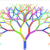# How to calculate the hours between start and finish times // Como calcular las horas comprendidas entre las horas de entradas y horas de salidas

432
1
12-16-2021 04:46 AMOccasional Contributor

Hello
For the realization of a survey for survey123 I need to calculate the hours between the hours of entry and exit, the problem occurs when it goes from 12:00 a.m. to 13:00 a.m. or from 23:59 p.m. to 0:00 a.m..
causes errors and these calculations are not correct.
Does anyone know what is the formula to enter?

#######################ESPAÑOL###############################################

Hola
Para la realización de una encuesta para survey123 necesito calcular las horas que transcurren entre las horas de entrada y salida, el problema ocurre cuando pasa de las 12:00 a.m. a la 13:00 a.m. o de 23:59 p.m. a 0:00 a.m.
provoca errores y esos cálculos no son correctos.
¿Alguien sabe cual es la formula que hay que introducir?

Saludos y gracias de antemano

Javier C. Martinez Prieto
Tags (5)
1 Solution

Accepted SolutionsbyEsri Regular Contributor

Are your entry and exit times entered using the time question type? If so, you can use something like the following to get the duration in minutes:

(decimal-time(\${exit_time}) - decimal-time(\${entry_time})) *24 *60

Note that both time questions are in the same 24-hour period, so for example, if you enter 10:00pm for the entry and 2:00am for the exit, the calculation will result in -1200 minutes (i.e. back in time 20 hours).

If you want your entry and exit times to cross from one day into the next, you could add the date to the value. One way to achieve this would be to use the dateTime question type instead for entry and exit, then use a calculation like:

(decimal-date-time(\${exit_datetime}) - decimal-date-time(\${entry_datetime})) *24 *60

If you prefer to use the time question type, one option could be to add 24 hours to the exit time, if that time is less than the entry time. Expression could be something like:

if(\${exit_time} > \${entry_time}, (decimal-time(\${exit_time}) - decimal-time(\${entry_time})) *24 * 60, ((decimal-time(\${exit_time}) - decimal-time(\${entry_time})) *24 * 60) + 1440)

One issue with this method is you would be relying on the user to enter the times correctly and making the assumption that they understand they're entering a time for the following day. It also wouldn't account for an exit time that was more than one day in the future (must be in the next 24 hours only). For this reason, the dateTime option is perhaps more reliable.

Note these examples are in minutes but you could drop the *60 to get hours, but you might need to use the round() function to get a suitable result.

For more help, see the Dates and Times sample XLSForm in Survey123 Connect, and check out the Dates and Time in Survey123 blog post.

Hope this helps,

Best, JimbyEsri Regular Contributor

Are your entry and exit times entered using the time question type? If so, you can use something like the following to get the duration in minutes:

(decimal-time(\${exit_time}) - decimal-time(\${entry_time})) *24 *60

Note that both time questions are in the same 24-hour period, so for example, if you enter 10:00pm for the entry and 2:00am for the exit, the calculation will result in -1200 minutes (i.e. back in time 20 hours).

If you want your entry and exit times to cross from one day into the next, you could add the date to the value. One way to achieve this would be to use the dateTime question type instead for entry and exit, then use a calculation like:

(decimal-date-time(\${exit_datetime}) - decimal-date-time(\${entry_datetime})) *24 *60

If you prefer to use the time question type, one option could be to add 24 hours to the exit time, if that time is less than the entry time. Expression could be something like:

if(\${exit_time} > \${entry_time}, (decimal-time(\${exit_time}) - decimal-time(\${entry_time})) *24 * 60, ((decimal-time(\${exit_time}) - decimal-time(\${entry_time})) *24 * 60) + 1440)

One issue with this method is you would be relying on the user to enter the times correctly and making the assumption that they understand they're entering a time for the following day. It also wouldn't account for an exit time that was more than one day in the future (must be in the next 24 hours only). For this reason, the dateTime option is perhaps more reliable.

Note these examples are in minutes but you could drop the *60 to get hours, but you might need to use the round() function to get a suitable result.

For more help, see the Dates and Times sample XLSForm in Survey123 Connect, and check out the Dates and Time in Survey123 blog post.

Hope this helps,

Best, Jim